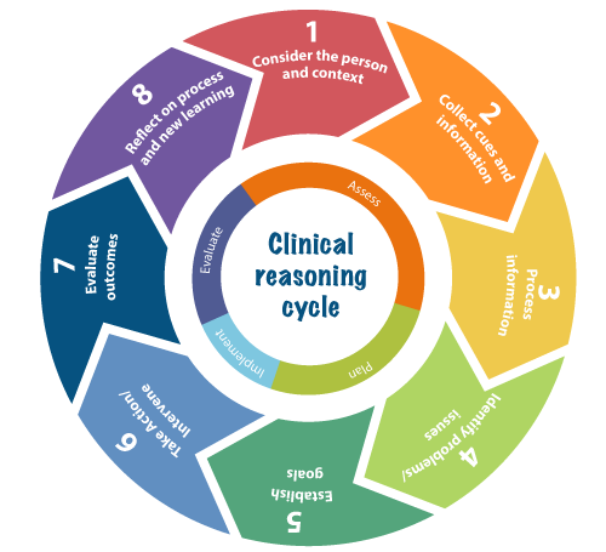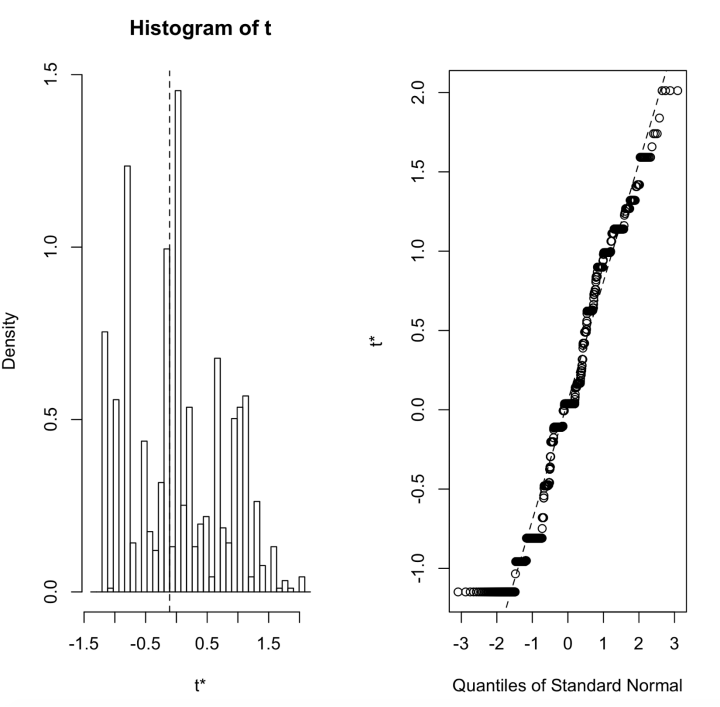# FIT2086 Studio 9 Supervised Machine Learning Methods Assessment AnswerPages: 4Words: 890

## Question :

Introduction

Studio 8 introduces you to several supervised machine learning techniques; in particular, you will look   at using decision trees for regression and classification, as well as nearest neighbours methods. To complete this Studio, you will need to install three packages: rpart, randomForest and kknn in R.

During your Studio session, your demonstrator will go through the answers with you, both on the board and on the projector as appropriate. Any questions you do not complete during the session should be completed out of class before the next Studio. Complete solutions will be released on the Friday after your Studio.

Decision Trees

In the first part of this Studio we will look at how to learn a basic decision trees from data, how to visualise/interpret the tree, and how to make predictions. We we will look at both continuous targets (regression trees) as well as categorical targets (classification trees). Begin by ensuring that the rpart package is loaded.

1. Load the  diabetes.train.csv  and  diabetes.test.csv  data into R. Use summary() to inspect your training data; you will see that it has 10 predictors, AGESEXBMIBP (blood pressure) and six blood serum measurements S1 through to S6; it also has a target variable Y, which is a measure of diabetes progression over a fixed period of time. The higher this value, the worse the diabetes progression.

2. Let us fit a decision tree to our training data. To do this, use

tree.diabetes rpart(Y ., diabetes.train)

which fits a decision tree to the data using some basic heuristics to decide when to stop growing the tree.

3. We can also explore the relationships between the diabetes progression outcome variable (Y) and the predictor variables that were used by the decision tree package. We will first do this by examining the decision tree in the console:

tree.diabetes

This  displays  the  tree  in  text  form.  The  asterisks  *  denote  the  terminal  (leaf)  nodes  of  the tree, and the nodes without asterisks are split nodes; the information contains which variables are split on, what the splits are, and for each leaf node, what the predicted value of is. How many leaf nodes are there in this tree? Which variables has the tree used to predict diabetes progression?

4. The output of the above command produces all the information related to the tree we have learned but can be hard to understand. It is easier to visualise the decision tree by using the plot() function to get a graphical representation of the relationships:

plot(tree.diabetes) text(tree.diabetes, digits=3)

This displays the tree, along with the various decision rules at each split node, and the predicted value of Y at each leaf. You may need to click the “Zoom” button above the plot in R Studio to get this picture displayed more clearly. Using this information answer the following questions:

1. What is the estimated average diabetes progression for individuals with BMI 28.0, blood pressure (BP) 96 and S6 = 110?
2. What is the estimated average diabetes progression for individuals with BMI = 20.1, S5 4.7 and S3 38?
3. Find the characteristics of the individuals with the worst (highest) predicted average dia- betes progression.
4. The rpart package provides a measure of importance for each variable. To access this type

tree.diabetes\$variable.importance

This reports the variables in order of importance, the importance being defined by the amount that they increase the goodness-of-fit of the tree to the data. Larger scores are better, though the numbers are themselves defined in terms of an arbitrary unit so it might be better to use

tree.diabetes\$variable.importance / max(tree.diabetes\$variable.importance)

which normalizes the importance scores so that they are relative to the importance of the most important predictor. Which three predictors are the most important?

1. We  can now test to see how well this tree predicts onto future data.  We  can use the  predict() function to get predictions for new data, and then calculate the root-mean squared error (RMSE):

sqrt(mean((predict(tree.diabetes, diabetes.test) - diabetes.test\$Y)^2))

How can we interpret this score?

2. The rpart package provides the ability to use cross-validation to try and “prune” the tree down, removing extra predictors and simplifying the tree without damaging the predictions too much (and potentially improving them). The code is a little involved, so I have included a wrapper function in the file wrappers.R; source this file to load the wrapper functions into memory. Then, to perform CV

cv = learn.tree.cv(Y ~.,data=diabetes.train,nfolds=10,m=1000)

The nfolds parameter tells the code how many different ways to break up the data, and a value of 10 is usually fine. The m parameter tells the code how many times to repeat the cross-validation process (randomly dividing the data up, training on some of the data, testing on the remaining data) – the higher this number is, the less the trees found by CV will vary from run to run, as  it reduces the random variability in the cross-validation scores – but the longer the training will take as we are doing more cross-validation tests. The cv object returned by learn.tree.cv() contains three entries. The cv\$cv.stats object contains the statistics of the cross-validation. We can visual this using:

plot.tree.cv(cv)

This shows the cross-validation score (y-axis) against the tree size in terms of number of leaf nodes (x-axis). The cv\$best.cp entry is the best value of the complexity parameter for our dataset, as estimated by CV, and can be passed to prune.rpart() to prune our tree down. The optimum number of leaf nodes is plotted in red.

1. The cv\$best.tree object contains the pruned tree, using cv\$best.cp as the pruning complexity parameter. Plot this tree, and compare it to the previous tree tree.diabetes. How do they compare
2. Has cross-validation removed any predictor variables from the original tree tree.diabetes?
3. What are the characteristics that predict the worst diabetes progression in this new tree?
4. What is the RMSE for this new tree cv\$best.tree on the test data?
5. We can now compare the performance of our decision tree to a standard linear model. Use the glmnet package to fit a linear model using the lasso, and calculate the RMSE for the fitted model:

lasso.fit = cv.glmnet.f(Y ~ ., data=diabetes.train) glmnet.tidy.coef(lasso.fit) sqrt(mean((predict.glmnet.f(lasso.fit,diabetes.test)-diabetes.test\$Y)^2))

How does the linear model compare in terms of which predictors it has chosen to use with the tree selected by CV?

Random Forests

A random forest is a collection of classification or regression trees that are grown by controlled, random splitting. Once grown, all the trees in a random forest are used to make predictions and to determine which predictors are associated with the outcome variable. However, the relationship between the predictors and the target is much more opaque than for a decision tree or linear model. In order to use random forests in R you must install and load the randomForest package.

First, use R to learn a random forest from the diabetes.train data set:

rf.diabetes = randomForest(Y ~ ., data=diabetes.train)

This trains a forest of decision trees on our data.

Unlike a single decision tree, a random forest is difficult to visualise and interpret as it consists of many hundreds or thousands of trees. After learning the random forest from the data, we can inspect the model by typing: rf.diabetes

This returns some basic information about the model, such as the percentage of variance ex- plained by the tree (roughly equivalent to 100 R2).

1. To see how well our random forest predicts onto our testing data we can use the predict()

function and calculate RMSE:,sqrt(mean((predict(rf.diabetes, diabetes.test) - diabetes.test\$Y)^2))

We can see that the random forest performs quite a bit better than our single best decision tree, and is basically the same as the linear model in this case.

2. So far we have been run the random forest package using the default settings for all parameters. Although the package randomForest has many interesting user-settable options (see the help for more details), the following three options are most useful for common use: ntree: Specifies the number of trees to grow. This should not be set to too small a number, to ensure that every input row gets predicted at least a few times (Default: 500)

• importance: Should importance of predictors be computed? (Default: FALSE) Let’s explore how we can use these options when analysing our diabetes data set.

rf.diabetes = randomForest(Y ~ ., data=diabetes.train, importance=TRUE, ntree=5000)

The number of trees in this example is set to 5000. In general, using more trees leads to improvements in prediction error. However, the computation complexity of the algorithm grows with the number of trees which means large forests can take a long time to learn and use for prediction. Calculate RMSE on the test data for this new random forest.

3. The option importance tells the random forest package that we wish to rank our predictor variables in terms of their strength of associat

Report

Question 1

Part 1

Number of leaves in best tree = 7

Variables used in best tree are: THAL, AGE, CP, EXANG, CA, CHOL

Part 2

Plot of best tree:According to this decision tree, most important features which decide about heart disease are THAL, AGE, CP, EXANG, CA, CHOL.

Following are the rules which decide whether a patient has disease or not

1. (THAL = Normal) and (Age < 54.5) à No
2. (THAL = Normal) and (Age >= 54.5) and (EXANG = Y) à Yes
3. (THAL = Normal) and (Age >= 54.5) and (EXAMG = N) and (CHOL < 304)à No
4. (THAL = Normal) and (Age >= 54.5) and (EXAMG = N) and (CHOL >= 304)à Yes
5. (THAL != Normal) and (CP = Asymptomatic) à Yes
6. (THAL != Normal) and (CP != Asymptomatic) and (CA < 0.5) à No
7. (THAL != Normal) and (CP != Asymptomatic) and (CA >= 0.5) à Yes

Part 3Tree plot with probability of each class at leaf node:Part 4

According to this tree,

(THAL != Normal) and (CP = Asymptomatic) à Yes has 0.8787 probability to have heart disease.

Part 5

Variables included by logistic regression model

THAL, CP, CA, EXANG, OLDPEAK.

Whereas tree model include following features THAL, CP, EXANG, CA, CHOL, AGE. CP has biggest coefficient in this logistic regression equation, so CP is one of the most important predictor in logistic regression

Part 6

Logistic regression equation

Z   =           (-0.8075472)                               +

(-0.8793322)*CPAtypical          +

(-1.4017135)*CPNonAnginal   +

(-2.6324294)*CPTypical             +

(1.3348209)*EXANGY                 +

(0.5519443)*OLDPEAK               +

(0.8486996)*CA                          +

(-0.9972506)*THALNormal       +

(0.8878904)*THALReversible.Defect

Y = 1/ 1+e-z

Part 7

Prediction statistics of treePrediction statistics logistic regressionAccuracy of d-tree is 84.84%, and accuracy of logistic regression is 86.36.% Whereas sensitivity(recall) of decision tree is better than logistic regression, and specificity(precision) of logistic regression is better than decision tree. So, in case of diagnostic test, once would prefer d-tree over logistic regression as it has higher recall i.e it has higher accuracy to catch positive cases.

Part 8

According to logistic regression model:

Probability of 45th example to be positive for heart disease is 0.71

According to d-tree model:

Probability of 45th example to be positive for heart disease is 0.3

Part 995% confidence interval for probability of having heart disease for patient in the 45th row in the test data is (0.39, 0.8983).

Probability of having heart disease for patient in the 45th row, according to the logistic regression model in part 1.8 is 0.71 and lies inside 95% confidence interval (0.39, 0.8983). Whereas probability of having heart disease for patient in the 45th row, according to the d-tree model in part 1.8 is 0.3 and it does not lie inside 95% confidence interval (0.39, 0.8983) computed for logistic regression. This means prediction for patient in 45th row is YES according to logistic regression but is NO according to d-tree.

Part 1095% Confidence interval for the classification accuracy of the logistic regression model using the predictors selected by BIC is (0.8182,0.8788).

Actual classification accuracy obtained on the testing data using the model you learned in Q1.6 is 86.36% and lies inside the 95% confidence interval (0.8182,0.8788)Question 2

Part 1aPart 1b

Plot for k = 2Plot for k = 5Plot for k = 10Plot for k = 25Part 1c

Visually we can see that estimated curve is closest to actual test curve for k = 5. For k = 2 and k = 10, the estimated curve is distinguishable from actual test curve, and the gap increase for k = 25.

So, rms for k = 5 will be minimal out the four cases here.

Part 2

Optimal value of k chosen by cross-validation method is 3. In question 2.1a, we have seen that mean-squared-error on test data is minimized at k = 4. This is possible as data used by cross-validation is training data, we can notice that optimal value of k using both the methods don’t differ much.

Part 3

Sensor measurement noise for test data will be equal to the difference between given intensity values and the predicted values (using k = 5). Variance of this difference is = 0.6859631

Part 4

Yes, for k = 5, knn method is able to achieve our aim of providing a smooth, low-noise estimate of background level as well as accurate estimation of the peaks.

According to me, knn is able to estimate the peaks well because this method learns the behaviour of neighbour points, if neighbour points in training data  have peak values, algorithms assigns higher values to test points as well.

Part 5

Plot using d-treeWe can see that d-tree is estimating the test data much poorly as compared to knn method. Mse of knn for k = 5 was 0.717139, and Mse of d-tree is 4.723485.

Part 6

Similarity between D-tree and Knn: Broadly both of these algorithms are trying to find the nearest neighbourhood of a point and then use mean to give the prediction.

Difference between D-tree and Knn: But the major difference between the two is the way of finding the neighbours. D-tree fid the neighbours by making splits of the form MZ > R (where R is a real number) Or MZ < R. But Knn method finds the neighbours by directly calculating the Euclidian distance.

The choice of getting nearest neighbours suits best for knn in this particular example, but for some other problem with higher dimension, d-tree generally works well.

Part 7Tags: